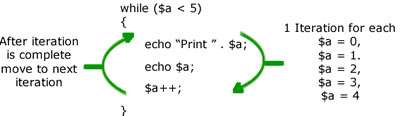# Difference between Recursion and Iteration

##### Key Difference: In programming, recursion can be explained by considering a recursive function. A recursive function is one which calls itself again to repeat the code. On the other hand, iteration is achieved by an iterative function which loops to repeat some section of the code.

In programming, recursion and iteration are both used to achieve repetitions. They refer to a process that is repeated numerous times. Recursion is based on an approach in which something refers to itself until a condition is met. A method is said to be recursive if it can call itself either directly or indirectly like –void name()

{

... name() ...

}

or

void name()

{

... game() ...

}

void game() {

... name() ...

}

For a successful recursion, one must keep in mind that every call made in the recursion process must simplify the computation. Recursion is achieved by defining a base case.

int factorial (int N)

{

if (N == 0) return 1;

else return (N*factorial(N-1));

}

In this example, recursion can easily be seen in the statement (N*factorial(N-1)), where it is calling the factorial function again. Recursion is very helpful as it helps in shortening of the code. However, the recursion is a little slow in performance.Iteration is based on loops. These loops refer to explicit iteration processes. For meeting the requirement of a loop, it must have some type of criteria that stops further iteration. However, if the loop-condition test never becomes false, then in that condition the occurrence of an infinite loop is inevitable. In this example, factorial is being determined by using the iteration process –

function factorial(n)

{

var loop, result;

result = 1;

for(loop=1;loop<=n;loop++)

{

result = result * loop;

}

return result;

}

In this example, looping is achieved by using integers from 1 to n, and loop<=n statement is used as a criteria to stop further looping. Thus, we can conclude that the same results can be achieved by using a recursion and iteration. However, they both are based on approaches that are a little different. Any recursive algorithm can also be written using iterations (loops).

Comparison between Recursion and Iteration:

 Recursion Iteration Definition Recursion refers to a recursive function in which it calls itself again to repeat the code. Iteration is achieved by an iterative function which loops to repeat some section of the code. Important point A base case needs to be determined A termination condition needs to be determined Performance Comparatively slow Comparatively fast Memory Usage Comparatively more Comparatively less Code Smaller Longer Infinite repetition Infinite recursion is capable of crashing the system Infinite looping consumes CPU cycles repeatedly Structure Selection Repetition Local variables Not required Required

Image Courtesy: zimbio.com, afterhoursprogramming.com